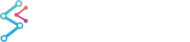﻿ Fibonacci Extension Drawing Tool | WPF Chart DocumentationSciChart WPF 2D Charts > The Trading Drawing Tools API > Fibonacci Extension Drawing Tool
Fibonacci Extension Drawing Tool

FibonacciExtensionAnnotation is an annotation useful for trading charts based on a Fibonacci Ratio.  It prorjects the Fibonacci Trable to a third (extended) point on the chart.

Note: The Drawing Tools API in SciChart.Charting.DrawingTools is available in the Enterprise and SDK Editions of SciChart onlyAs the FibonacciExtensionAnnotation is a CompositeAnnotation (annotation that consists of a number of other annotations) it has no special properties, only common properties of usual annotations.

The FibonacciRetracementAnnotation consists of:

• Two lines – which are actually LineAnnotations
• The first is a trendline
• The second is used for projecting the FibonnaciTable to some future point
• FibonacciTable – which is a CompositeAnnotation that consists of multiple of FibonacciRatioLine annotations.
• FibonacciRatioLine has all properties of HorizontalLineAnnotation and one special property, called “LineLevel”. This property corresponds to a level value(in percentage).

## Declaring a FibonacciExtensionAnnotation in XAML

To declare a FibonacciRetracementAnnotation in XAML you have to declare the annotation and then declare its InitialBasePoints collection that is actually collection of ComparablePoint objects. ComparablePoint stores X and Y values that are used for placing annotation point.

Declaring a FibonnacciRetracementAnnotation in XAML
Copy Code
```<!-- XAxis, YAxis, RenderableSeries omitted for brevity -->
<s:SciChartSurface.Annotations>

<!--Declare FibonacciExtensionAnnotation-->
<s:FibonacciExtensionAnnotation IsEditable="True">

<!-- Declare InitialBasePoints collection that is used for
creating annotation from XAML.
InitialBasePoints is actually observable collection of
ComparablePoint-s. ComparablePoint stores X and Y point Value.
-->
<s:FibonacciExtensionAnnotation.InitialBasePoints>
<!-- Placing first point -->
<s:ComparablePoint X="2"  Y="4"/>
<!-- Placing second point -->
<s:ComparablePoint X="9"  Y="-4"/>
<!-- Placing third point -->
<s:ComparablePoint X="9"  Y="-4"/>

</s:FibonacciExtensionAnnotation.InitialBasePoints>
</s:FibonacciExtensionAnnotation>
</s:SciChartSurface.Annotations>
```

Please Note that the FibonacciExtensionAnnotation has limit of three base points. As you can see here, you just have to set this three points for FibonacciExtensionAnnotation: [X1=0; Y1=0], [X2=6; Y2=6] and [X3=10; Y3=2].

## Declaring a FibonacciExtensionAnnotation in Code-Behind

To add a FibonacciExtensionAnnotation in code, simply use the following C# code:

Declaring a FibonnacciExtensionAnnotation in Code
Copy Code
```// Create a SciChartSurface. XAxis, YAxis omitted for brevity
var sciChartSurface = new SciChartSurface();
// Create a FibonacciExtensionAnnotation.
var fibonacciExtension = new FibonacciExtensionAnnotation();
// FibonacciExtensionAnnotation should be added to
//SciChartSurface.Annotation collection first, to make SetBasePoint() method
//works correctly
// Set first point of FibonacciExtensionAnnotation
fibonacciExtension.SetBasePoint(0, 0);

// Set second point of FibonacciExtensionAnnotation
fibonacciExtension.SetBasePoint(6, 6);
// Set third point of FibonacciExtensionAnnotation
fibonacciExtension.SetBasePoint(10, 2);
```

## Declaring a FibonacciExtensionAnnotation in MVVM

To add a FibonacciExtensionAnnotation via MVVM please follow the instructions for adding annotations with MVVM: Declaring Annotations in MVVM with the AnnotationsBinding Markup Extension

To add a FibonacciExtensionAnnotation via a ViewModel you have to use SciChart markup extension called AnnotationsBinding,

The AnnotationsBinding Markup Extension
Copy Code
```<s:SciChartSurface Annotations="{s:AnnotationsBinding Annotations}">
</s:SciChartSurface>
```

Now you need to create collection for Annotations in ViewModel. Then create and add a FibonacciExtensionAnnotationViewModel to that collection of Annotations like this:

Declaring a FibonnacciExtensionAnnotation in a ViewModel
Copy Code
```public class MyViewModel : INotifyPropertyChanged
{
public ObservableCollection<IAnnotationViewModel> Annotations { get; }

public void Foo()
{
// Create a FibonacciExtensionAnnotation.
fibonacciExtension = new FibonacciExtensionAnnotationViewModel();
// FibonacciExtensionAnnotation should be added to
//SciChartSurface.Annotation collection first, to make SetBasePoint() method
//works correctly
// Set first point of FibonacciExtensionAnnotation
fibonacciExtension.SetBasePoint(0, 0);

// Set second point of FibonacciExtensionAnnotation
fibonacciExtension.SetBasePoint(6, 6);
// Set third point of FibonacciExtensionAnnotation
fibonacciExtension.SetBasePoint(10, 2);
}
}
```